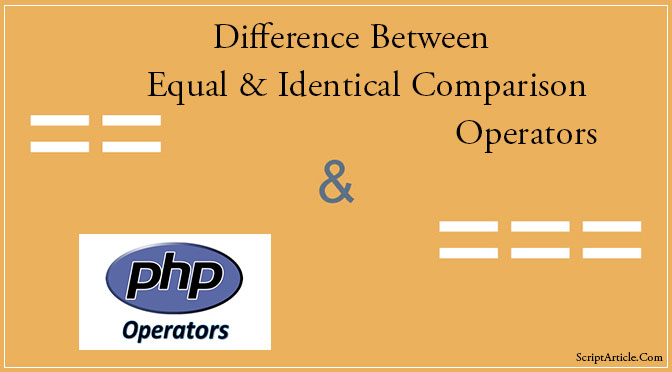# Equal and Identical comparison operators in PHP / == (equal) or === (identical)Equal and Identical comparison operators in PHP

When comparing values in PHP for equality you can use either the == (equal) operator or the === (identical) operator. Do you know, what is the difference?

Very simple the == operator just checks to see if the left and right values are equal. But, the === operator (note the extra ‘=’) actually checks to see if the left and right values are equal, and also checks to see if they are of the same variable type (like whether they are both boolean, ints, etc.).

Example of when you need to use the === operator in PHP

[php]

<?php

if ( strpos( \$yourString, ‘searchMe’ ) == false ) {
}

//Good code
if ( strpos( \$ yourString, ‘searchMe’ ) === false ) {
}

?>

[/php]

You can now see that ‘==’ check values only but ‘===’ check values as well as the data type of vales also.

The operator == casts between two different types if they are different, while the === operator performs a ‘typesafe comparison’. That means that it will only return true if both operands have the same type and the same value.

Hope it will make sense?

## One thought on “Equal and Identical comparison operators in PHP / == (equal) or === (identical)”

1.bhrnvmlkoh says:

I like your site. Your texts are interesting. I got here by mistake as well as I started reading. I became interested in the subject and I am thinking it I could use your texts on my paper, of course with the quotation. Please contact with me, thank you.